# A lemma to identify dvrs

In this post I will prove a commutative algebra lemma, which is proved in page 7 of Serre’s Local Fields. This lemma is useful if you find a ring in the wild and want to know that it’s a discrete valuation ring.

Proposition: Letbe a noetherian local domain where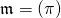and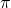is not nilpotent. Then.

Proof: Suppose. Then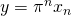for all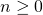. So for all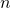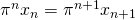. Since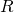is a domain,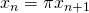.

Therefore consider the ascending chain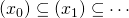. This eventually stabilizes for high enoughsinceis noetherian, so for some,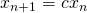. Thus, so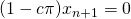. Butis a unit, so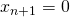, so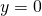.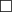This theorem holds more generally even ifis not assumed to be a domain, but the proof is more complicated (but still among the same lines).

Proposition: Letbe a noetherian local ring whereandis not nilpotent. Then.

Proof: Let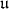be the ideal of elements that kill some power of. We will use variables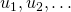to refer to elements of. Sinceis noetherian,must be finitely generated, so all elements ofkill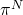for some fixed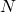.

Now suppose., so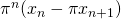. Thus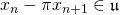.

Consider the ascending chain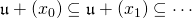. Sinceis noetherian it must eventually stablize, so for some,can be written as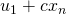. But recall that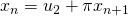. So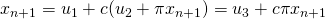so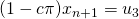.is a unit since, andis local, so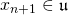. If we forceto be large enough to surpass, then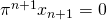, so.# Hilbert’s Basis Theorem

Here is a proof of Hilbert’s Basis Theorem I thought of last night.

Letbe a noetherian ring. Consider an ideal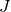in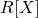. Let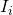be the ideal ingenerated by the leading coefficients of the polynomials of degreein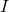. Notice that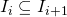, since if,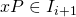, and it has the same leading coefficient. Thus we have an ascending chain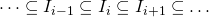, which must terminate, sinceis noetherian. Suppose it terminates at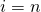, so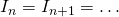.

Now for eachchoose a finite set of generators (which we can do sinceis noetherian). For each generator, choose a representative polynomial inwith that leading coefficient. This gives us a finite collection polynomials: define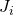to be the ideal of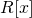generated by these polynomials.

Let. I claim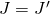. Assume for the sake of contradiction that there is a polynomial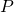of minimal degree (say) which is inbut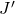. If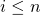, there is an element of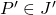with the same leading coefficient, so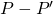is not inbut has degree smaller than: contradiction. Ifis of degree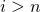, then there is an element of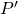of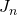which has the same leading coefficient as. Thus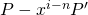is of degree smaller thanbut is not in: contradiction.

Thus. Sinceis therefore finitely generated, this provesis noetherian.